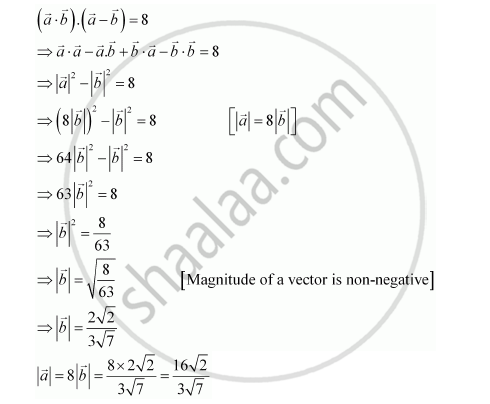Share

# Find |Veca| and |Vecb|, If (Veca + Vecb).(Veca -vecb) = 8 and |Veca| = 8|Vecb| - Mathematics

#### Question

Find |veca| and |vecb|, if (veca + vecb).(veca -vecb) = 8 and |veca| = 8|vecb|

#### SolutionIs there an error in this question or solution?